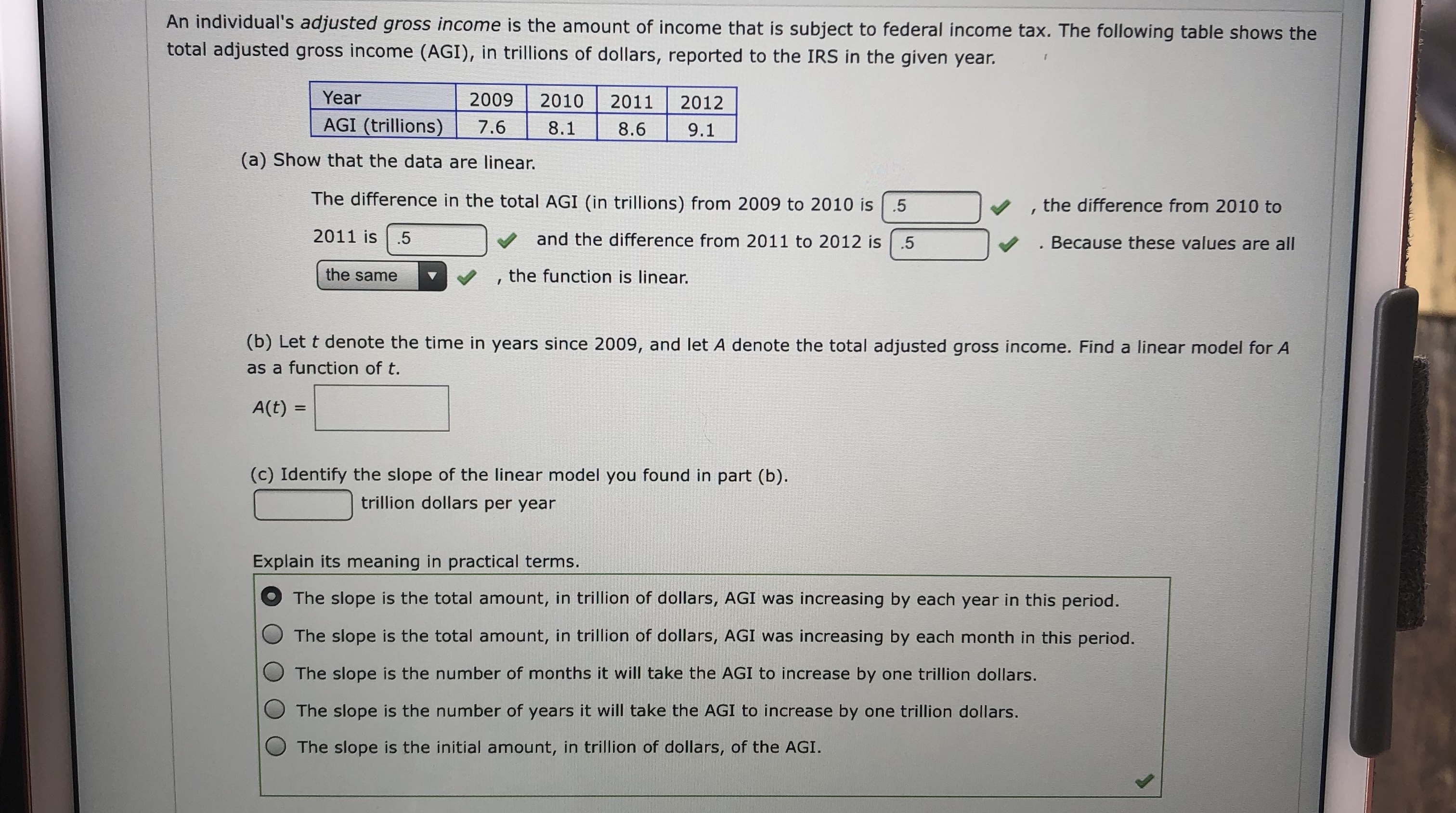# An individual's adjusted gross income is the amount of income that is subject to federal income tax. The following table shows thetotal adjusted gross income (AGI), in trillions of dollars, reported to the IRS in the given year.Year2009201020112012AGI (trillions)7.68.18.69.1(a) Show that the data are linear.The difference in the total AGI (in trillions) from 2009 to 2010 is.5the difference from 2010 to2011 isand the difference from 2011 to 2012 is .5. Because these values are allthe samethe function is linear.(b) Let t denote the time in years since 2009, and let A denote the total adjusted gross income. Find a linear model for Aas a function of t.A(t)(c) Identify the slope of the linear model you found in part (b)trillion dollars per yearExplain its meaning in practical terms.The slope is the total amount, in trillion of dollars, AGI was increasing by each year in this period.The slope is the total amount, in trillion of dollars, AGI was increasing by each month in this period.The slope is the number of months it will take the AGI to increase by one trillion dollars.OThe slope is the number of years it will take the AGI to increase by one trillion dollars.The slope is the initial amount, in trillion of dollars, of the AGI.

Question
199 views

What are the blanks?help_outlineImage TranscriptioncloseAn individual's adjusted gross income is the amount of income that is subject to federal income tax. The following table shows the total adjusted gross income (AGI), in trillions of dollars, reported to the IRS in the given year. Year 2009 2010 2011 2012 AGI (trillions) 7.6 8.1 8.6 9.1 (a) Show that the data are linear. The difference in the total AGI (in trillions) from 2009 to 2010 is.5 the difference from 2010 to 2011 is and the difference from 2011 to 2012 is .5 . Because these values are all the same the function is linear. (b) Let t denote the time in years since 2009, and let A denote the total adjusted gross income. Find a linear model for A as a function of t. A(t) (c) Identify the slope of the linear model you found in part (b) trillion dollars per year Explain its meaning in practical terms. The slope is the total amount, in trillion of dollars, AGI was increasing by each year in this period. The slope is the total amount, in trillion of dollars, AGI was increasing by each month in this period. The slope is the number of months it will take the AGI to increase by one trillion dollars. OThe slope is the number of years it will take the AGI to increase by one trillion dollars. The slope is the initial amount, in trillion of dollars, of the AGI. fullscreen
check_circle

Step 1

b) Let t denote the time in years since 2009 and D den...

### Want to see the full answer?

See Solution

#### Want to see this answer and more?

Solutions are written by subject experts who are available 24/7. Questions are typically answered within 1 hour.*

See Solution
*Response times may vary by subject and question.
Tagged in

### Algebra